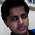### Find Fixed Point (x[i]=i) in an Array

Source: Asked in an Amazon interview to a friend

Problem:

Given an array of size n, x[0 .. n-1] of integers sorted into ascending order with no duplicates, find an array item that is also its index, so that x[i] = i.

For example, x = 3 in the array shown below:

i           0 1 2 3 4 5
x[i]     -3 0 1 3 5 7

Disclaimer:
Yes, its a very easy problem, as far as algorithm is concerned. Its here for people preparing for interviews to see if they can write code.

1.Binary search or better?

2.Once you know that f(i) = x[i]-i is an non-decreasing sequence, it's a simple binary search

3.Binary search!!

If x[i] == i, return
if x[i] < i, move right
if x[i] > i, move left

4.[x for i,x in enumerate(a) if i==x]

5.Do binary search. Will take O(log n)

6.int FixedPointBinarySearch(int a[],int n)
{
int mid=(n)/2;
if(n==1)
return 0;
if(a[mid]==mid)
return mid;
else if(a[mid]<mid)
return mid+FixedPointBinarySearch(a+mid+1,n-mid-1);
else
return FixedPointBinarySearch(a,n-mid);
}

7.idx = 0
for spread = 0 to max_number(array)
if spread = idx then msgbox "match"
idx = idx + 1

8.public int findI(int[] a){
int n = a.length;
if(a>n || a[n-1]<0){
return -1;
}
int temp = n/2;
while(true){
if(temp > n-1)
return -1;
if(a[temp]==temp)
return temp;
else if(a[temp]>temp)
temp = temp/2;
else
temp = temp + temp/2;
}
}

return i if found and -1 otherwise!!!

9.def fixpoint(a):
x,y = 0,len(a)
while x+1 != y:
h = x + ((y - x) >> 1)
if a[h] <= h:
x = h
else:
y = h
return a[x] == x

10.Use bin search
1.if mid value mid then search in left part.
3.return mid if found else -1.